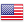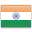Grade "A+" Accredited by NAAC with a CGPA of 3.46
Grade "A+" Accredited by NAAC with a CGPA of 3.46

# Combinatorial Optimization

Course ID
BHCS 15C
Level
Program
B.Sc. CS (Hons.)
Semester
Fifth
Credits
6.0
Paper Type
DSE - 1
Method
Lecture & Tutorial

## Unique Paper Code: Update Awaited

This course is designed to introduce the fundamentals of combinatorial optimization to the students in terms of both theory and applications, so as to equip them to explore the more advanced areas of convex and non-convex optimizations.

## Learning Outcomes:

At the end of the course, students should be able to:

• Model problems using linear and integer programs.
• Apply polyhedral analysis to develop algorithms for optimization problems.
• Use the concept of duality for design of algorithms.

## Course Contents

Unit 1
Unit 2
Unit 3
Unit 4

Unit 1

Introduction to Combinatorial Optimization Problems, Linear and Integer Programs: Formulation, understanding integer programs, computational complexities of IP vs LP, using LP to find optimal or approximate integral solutions, concept of integrality gap.

Unit 2

Theory of Linear Programming and Algorithmic Perspective to Simplex Method:: standard vs. equational form, basic feasible solutions, convexity and convex polyhedra, correspondence between vertices and basic feasible solutions, geometry of Simplex algorithm, exception handling (unboundedness, degeneracy, infeasibility), Simplex algorithm, avoiding cycles.

Unit 3

Primal-Dual Algorithms: interpretation of dual, optimality conditions for primal and dual, weak and strong duality, complementary slackness, primal-dual algorithm for the shortest path problem.

Unit 4

Network Flows: linear programming formulations for network flows and bipartite matching, totally unimodular matrices integral polyhedral.

#### Text Books

Matousek & Gartner (2007). Understanding and Using Linear Programming. Springer.

Papadimitriou, C.H. & Steiglitz, K. (1998). Combinatorial Optimization: Algorithms and complexity. Dover Publications.

Bazaraa, M.S., Jarvis, J.J., & and Sherali, H.D.(2008). Linear Programming and Network Flows. 2nd edition. Wiley.
Korte, B., & Vygen, J. (2006). Combinatorial Optimization. 5th edition. Springer.

#### Teaching Learning Process

Use of ICT tools in conjunction with traditional class room teaching methods
Interactive sessions
Class discussions

#### Assessment Methods

Written tests, assignments, quizzes, presentations as announced by the instructor in the class.

#### Keywords

Optimization problems, linear programming, integer programming, duality, network flow problems.

Disclaimer: Details on this page are subject to change as per University of Delhi guidelines. For latest update in this regard please refer to the University of Delhi website here.Englishहिन्दी